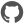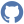# PositionMatrix

Namespace: GibbsSampling

Consists of functions to work with Position-Frequancy-, -Probability- and -WeightMatrices.

## Nested types and modules

 Type Description PositionFrequencyMatrix Matrix with fixed positions for nucleotides and amino acids with default value of 0. PositionProbabilityMatrix Matrix with fixed positions for nucleotides and amino acids with default value of 0. . PositionWeightMatrix

### Functions and values

 Function or value Description ``` calculateSegmentScoreBy (...) ``` Signature: pwMatrix:PositionWeightMatrix -> bioItems:(type) -> float Type parameters: '?7996Calculate the score of the given sequence based on the Probabilities of the PositionWeightMatrix. ``` createPFMOf source ``` Signature: source:(type) -> PositionFrequencyMatrix Type parameters: '?7976Create PositionFrequencyMatrix based on BioArray. ``` createPositionWeightMatrix (...) ``` Signature: alphabet:'?7994 [] -> pcv:ProbabilityCompositeVector -> ppMatrix:PositionProbabilityMatrix -> PositionWeightMatrix Type parameters: '?7994Create PositionWeightMatrix based on ProbabilityCompositeVector and PositionProbabilityMatrix. ``` createPPMOf positionFrequencyMatrix ``` Signature: positionFrequencyMatrix:PositionFrequencyMatrix -> PositionProbabilityMatrixCreate new PositionWeightMatrix based on existing PositionFrequencyMatrix. The counts of each position of each element are transformed to floats. ``` fusePositionFrequencyMatrices (...) ``` Signature: motiveLength:int -> countMatrices:PositionFrequencyMatrix [] -> PositionFrequencyMatrixCreate new PositionFrequencyMatrix based on the sum of the positions of an array of Countmatrices. ``` getBestInformationContent item ``` Signature: item:(float * int) [] [] -> (float * int) []Finds the best information content in an array of arrays of PWMSs and positions. ``` increaseInPlacePFM (...) ``` Signature: pos:int -> bioItem:'a -> positionFrequencyMatrix:PositionFrequencyMatrix -> PositionFrequencyMatrix Type parameters: 'aIncrease counter of PositionFrequencyMatrix at fixed position by 1. ``` increaseInPlacePFMBy (...) ``` Signature: pos:int -> bioItem:'a -> n:int -> positionFrequencyMatrix:PositionFrequencyMatrix -> PositionFrequencyMatrix Type parameters: 'aIncrease counter of PositionFrequencyMatrix at fixed position by n. ``` increaseInPlacePPM (...) ``` Signature: pos:int -> bioItem:'a -> positionProbabilityMatrix:PositionProbabilityMatrix -> PositionProbabilityMatrix Type parameters: 'aIncrease counter of PositionProbabilityMatrix at fixed position by 1. ``` increaseInPlacePPMBy (...) ``` Signature: pos:int -> bioItem:'a -> n:float -> positionProbabilityMatrix:PositionProbabilityMatrix -> PositionProbabilityMatrix Type parameters: 'aIncrease counter of PositionProbabilityMatrix at fixed position by n. ``` increaseInPlacePWM (...) ``` Signature: pos:int -> bioItem:'a -> positionWeightMatrix:PositionWeightMatrix -> PositionWeightMatrix Type parameters: 'aIncrease counter of PositionWeightMatrix at fixed position by 1. ``` increaseInPlacePWMBy (...) ``` Signature: bioItem:'a -> pos:int -> n:float -> positionWeightMatrix:PositionWeightMatrix -> PositionWeightMatrix Type parameters: 'a``` normalizePPM (...) ``` Signature: sourceCount:int -> alphabet:'?7986 [] -> pseudoCount:float -> positionFrequencyMatrix:PositionProbabilityMatrix -> PositionProbabilityMatrix Type parameters: '?7986The counts of each position of each element are transformed to floats.